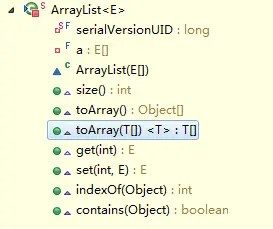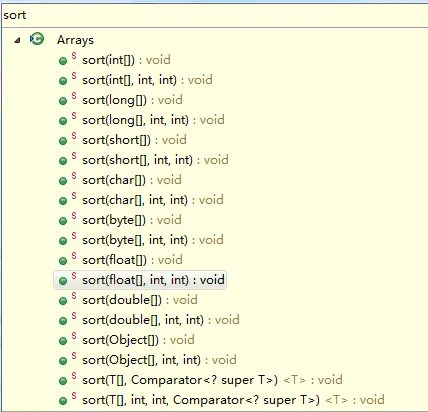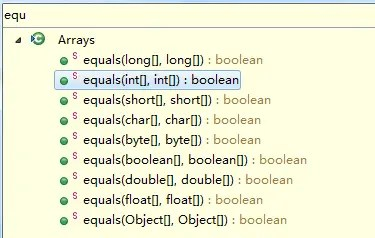# JDK1.8 source code (4)-java.util.Arrays class

Posted May 30, 20207 min read

The java.util.Arrays class is a tool class provided by JDK, used to process various methods of arrays, and each method is basically a static method, which can be directly called by the class name Arrays.

### 1. asList

``````    public static <T> List <T> asList(T ... a) {
return new ArrayList <>(a);
}``````

Returns a fixed-size list supported by the specified array.

Note:The ArrayList returned by this method is not our commonly used collection class java.util.ArrayList. ArrayList here is an internal class of Arrays java.util.Arrays.ArrayList. This inner class has the following properties and methods:``````private static class ArrayList <E> extends AbstractList <E>
implements RandomAccess, java.io.Serializable
{
private static final long serialVersionUID = -2764017481108945198L;
private final E []a;

ArrayList(E []array) {
if(array == null)
throw new NullPointerException();
a = array;
}

public int size() {
return a.length;
}

public Object []toArray() {
return a.clone();
}

public <T> T []toArray(T []a) {
int size = size();
if(a.length <size)
return Arrays.copyOf(this.a, size,
(Class <? Extends T []>) a.getClass());
System.arraycopy(this.a, 0, a, 0, size);
if(a.length> size)
a [size]= null;
return a;
}

public E get(int index) {
return a [index];
}

public E set(int index, E element) {
E oldValue = a [index];
a [index]= element;
return oldValue;
}

public int indexOf(Object o) {
if(o == null) {
for(int i = 0; i <a.length; i ++)
if(a [i]== null)
return i;
} else {
for(int i = 0; i <a.length; i ++)
if(o.equals(a [i]))
return i;
}
return -1;
}

public boolean contains(Object o) {
return indexOf(o)! = -1;
}
}``````

** The returned ArrayList array is a fixed-length list, we can only view or modify it, but cannot add or delete it**

Through the source code, we found that this class does not have add() or remove() methods. If it is added or deleted, the corresponding method of its parent class AbstractList will be called, and the method of tracing the parent class will eventually throw UnsupportedOperationException abnormal. as follows:

`````` String []str = {"a", "b", "c"};
List <String> listStr = Arrays.asList(str);
listStr.set(1, "e"); //Can be modified
System.out.println(listStr.toString()); //[a, e, c]** , the difference between the reference type array and the basic type array**

``````String []str = {"a", "b", "c"};
List listStr = Arrays.asList(str);
System.out.println(listStr.size()); //3

int []i = {1,2,3};
List listI = Arrays.asList(i);
System.out.println(listI.size()); //1``````

The first result of the above listStr.size() == 3, and the second listI.size() == 1. Why is this?

Look at the source code. In Arrays.asList, the method is declared as List asList(T ... a). This method receives a variable parameter, and this variable parameter type is used as a generic parameter. We know that basic data types cannot be used as generic parameters, but arrays are reference types, so arrays can be generalized, so int \ []is the entire parameter type, not int as the parameter type.

So the generalization of the above method should be:

``````String []str = {"a", "b", "c"};
List <String> listStr = Arrays.asList(str);
System.out.println(listStr.size()); //3

int []i = {1,2,3};
List <int []> listI = Arrays.asList(i); //Note that the List parameter is int []instead of int
System.out.println(listI.size()); //1

Integer []in = {1,2,3};
List <Integer> listIn = Arrays.asList(in); //Here the parameter is the int wrapper class Integer, so the collection length is 3
System.out.println(listIn.size()); //3``````

** The elements in the returned list ArrayList are all references, not independent objects**

``````String []str = {"a", "b", "c"};
List <String> listStr = Arrays.asList(str);
//Before performing the update operation
System.out.println(Arrays.toString(str)); //[a, b, c]
listStr.set(0, "d"); //Change the first element a to d
//After performing the update operation
System.out.println(Arrays.toString(str)); //[d, b, c]``````

The Arrays.toString() method here is to print the contents of the array, which will be introduced later. We look at modifying the content of the collection, the content of the original array has also changed, so the reference type is passed in here.

** Given the array data, how to quickly obtain a list that can be added, deleted, modified and checked?**

`````` String []str = {"a", "b", "c"};
List <String> listStr = new ArrayList <>(Arrays.asList(str));
System.out.println(listStr.size()); //4``````

Here we will explain in detail behind the ArrayList collection class. At present, you only need to know that there is such a usage.

** , Arrays.asList() method usage scenario**

Arrays tool class provides a method asList, which can be used to convert a variable-length parameter or array into a List. However, the length of the generated List is fixed; it can be modified(for example, to modify an element at a certain position); it cannot perform operations that affect the length(such as add, remove, etc.), otherwise it will throw an UnsupportedOperationException.

So Arrays.asList is more suitable for those scenarios that already have array data or some elements, and need to quickly build a List, which is only used for reading operations, without adding or deleting operations.

### 2.sort

This method is used for array sorting. There are a series of overloaded methods of this method in the Arrays class, which can sort 7 basic data types, including byte, char, double, float, int, long, short, etc., There is also the Object type(implementing the Comparable interface), and the Comparator.** , array of basic types**

Here we take int \ []as an example:

`````` int []num = {1,3,8,5,2,4,6,7};
Arrays.sort(num);
System.out.println(Arrays.toString(num)); //[1, 2, 3, 4, 5, 6, 7, 8]``````

By calling the sort(int \ []a) method, the original array is arranged in ascending order. Let's take a look at the source code to see how sorting is implemented:

``````    public static void sort(int []a) {
DualPivotQuicksort.sort(a, 0, a.length-1, null, 0, 0);
}``````

Call the DualPivotQuicksort.sort method inside the Arrays.sort method. The source code of this method is very long. Various algorithms are used to divide the length of the array, including quick sort, insert sort, and bubble sort. Detailed source code can refer to this blog.

** , Object type array**

Sorting of this type of array can implement the Comparable interface, and override the compareTo method to sort.

`````` String []str = {"a", "f", "c", "d"};
Arrays.sort(str);
System.out.println(Arrays.toString(str)); //[a, c, d, f]``````

The String type implements the Comparable interface, and the internal compareTo method compares according to dictionary codes.

** If the Comparable interface is not implemented, sorting can be achieved through the Comparator**

``````Person []p = new Person []{new Person("zhangsan", 22), new Person("wangwu", 11), new Person("lisi", 33)};
Arrays.sort(p, new Comparator <Person>() {
@Override
public int compare(Person o1, Person o2) {
if(o1 == null || o2 == null) {
return 0;
}
return o1.getPage()-o2.getPage();
}
});
System.out.println(Arrays.toString(p));``````

### 3, binarySearch

Use dichotomy to find an element in an array. This method, like the sort method, is applicable to various basic data types and objects.

Note:The dichotomy is to search for and ordered arrays(such as Arrays.sort() to sort first, and then call this method to find). Find the element and return the subscript, otherwise return -1

Examples:

`````` int []num = {1,3,8,5,2,4,6,7};
Arrays.sort(num);
System.out.println(Arrays.toString(num)); //[1, 2, 3, 4, 5, 6, 7, 8]
System.out.println(Arrays.binarySearch(num, 2)); //Return the index of the element 1``````

Specific source code implementation:

``````public static int binarySearch(int []a, int key) {
return binarySearch0(a, 0, a.length, key);
}
private static int binarySearch0(int []a, int fromIndex, int toIndex, int key) {
int low = fromIndex;
int high = toIndex-1;

while(low <= high) {
int mid =(low + high) >>> 1; //Take the middle value subscript
int midVal = a [mid]; //Take the middle value

if(midVal <key)
low = mid + 1;
else if(midVal> key)
high = mid-1;
else
return mid;
}
return-(low + 1);
}``````

### 4, copyOf

Copy array elements. The bottom layer is implemented with System.arraycopy(), which is a native method.

``````    public static native void arraycopy(Object src, int srcPos,
Object dest, int destPos,
int length);``````

Src:source array

SrcPos:the starting position of the source array to be copied

Dest:array of destinations

DestPos:the starting position of the destination array

Length:the length of the copy

Note:Both src and dest must be arrays of the same type or conversion type.

``````int []num1 = {1,2,3};
int []num2 = new int ;
System.arraycopy(num1, 0, num2, 0, num1.length);
System.out.println(Arrays.toString(num2)); //[1, 2, 3]

/**
* @param original source array
* @param newLength //Return the length of the new array
* @return
* /
public static int []copyOf(int []original, int newLength) {
int []copy = new int [newLength];
System.arraycopy(original, 0, copy, 0,
Math.min(original.length, newLength));
return copy;
}``````

### 5, equals and deepEquals

** , equals**

Equals is used to compare whether each element at the corresponding position in two arrays is equal.Eight basic data types and objects can be compared.

Let's take a look at the source code implementation of the int array comparison:

``````public static boolean equals(int []a, int []a2) {
if(a == a2) //The array references are equal, then the elements inside must be equal
return true;
if(a == null || a2 == null) //One of the two arrays is null, both return false
return false;

int length = a.length;
if(a2.length! = length) //The length of the two arrays are not equal, return false
return false;

for(int i = 0; i <length; i ++) //compare each element in the array in sequence through the for loop
if(a [i]! = a2 [i])
return false;

return true;
}``````

Looking at the comparison of the object array:

``````public static boolean equals(Object []a, Object []a2) {
if(a == a2)
return true;
if(a == null || a2 == null)
return false;

int length = a.length;
if(a2.length! = length)
return false;

for(int i = 0; i <length; i ++) {
Object o1 = a [i];
Object o2 = a2 [i];
if(!(o1 == null? o2 == null:o1.equals(o2)))
return false;
}

return true;
}``````

Is basically judged by equals.

** , deepEquals**

Is also used to compare whether the elements of two arrays are equal, but deepEquals can compare multi-dimensional arrays, and it is a nested array of any level.

``````         String [][]name1 = {{"G", "a", "o"}, {"H", "u", "a", "n"}, {"j", "i", " e "}};
String [][]name2 = {{"G", "a", "o"}, {"H", "u", "a", "n"}, {"j", "i", " e "}};
System.out.println(Arrays.equals(name1, name2)); //false
System.out.println(Arrays.deepEquals(name1, name2)); //true``````

### 6, fill

This series of methods is used to assign values to arrays and can specify a range of assignments.

``````    //Assign val to all elements of a array
public static void fill(int []a, int val) {
for(int i = 0, len = a.length; i <len; i ++)
a [i]= val;
}

//The subscripts starting with fromIndex and the subscripts ending with toIndex-1 are all assigned the value val, left closed and right open
public static void fill(int []a, int fromIndex, int toIndex, int val) {
rangeCheck(a.length, fromIndex, toIndex); //Judge whether the range is reasonable
for(int i = fromIndex; i <toIndex; i ++)
a [i]= val;
}``````

### 7, toString and deepToString

ToString is used to print the elements of a one-dimensional array, and deepToString is used to print multiple levels of nested array elements.

``````public static String toString(int []a) {
if(a == null)
return "null";
int iMax = a.length-1;
if(iMax == -1)
return "[]";

StringBuilder b = new StringBuilder();
b.append('[');
for(int i = 0;; i ++) {
b.append(a [i]);
if(i == iMax)
return b.append(']'). toString();
b.append(",");
}
}``````

This series of tutorials is continuously updated, you can search for "IT Coke" on WeChat for the first time. Reply "E-book" has the book material that I specially screened for you